# Signing a graph to have small magnitude eigenvalues

 Importance: Medium ✭✭
 Author(s): Bilu, Yonatan Linial, Nathan
 Subject: Graph Theory
 Keywords: eigenvalue expander Ramanujan graph signed graph signing
 Recomm. for undergrads: no
 Posted by: mdevos on: March 24th, 2013
Conjecture   If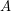is the adjacency matrix of a-regular graph, then there is a symmetric signing of(i.e. replace someentries by) so that the resulting matrix has all eigenvalues of magnitude at most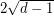.

A graphis a-lift of a graphif there is a-to-mapwhich is locally injective in the sense that the restriction ofto the neighbourhood of every vertex is an injection. We can construct a random-lift ofwith vertex set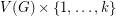by adding a (uniformly chosen) random matching between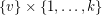andwhenever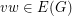. Ifis a-lift of, then every eigenvalue ofwill also be an eigenvalue of, but in additionwill have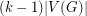new eigenvalues. There has been considerable interest and investigation into the behaviour of these new eigenvalues for a random-lift, since it is expected that they should generally be small in magnitude. In particular, ifis a Ramanujan graph (a-regular graph for which all nontrivial eigenvalues are at most) it may be possible to construct a new Ramanujan graph by taking a suitable-lift of. A series of increasingly strong results have shown that a random-lift of a-regular Ramanujan graph will have all new eigenvalues at most(Friedman [F]),(Linial and Pruder [LP]) and(Lubetzky, Sudakov, and Vu [LSV]).

An interesting paper of Bilu and Linial [BL] investigates 2-lifts of graphs. Letbe a graph and letbe a 2-lift ofwith vertex setas above. Every eigenvector ofextends naturally to an eigenvector ofwhich is constant on each fiber (set of the form). Thus, we may assume that all of the new eigenvalues are associated with eigenvectors which sum to zero on each fiber. So, each of these new eigenvectors is completely determined by its behaviour on. Now we assign a signatureto each edge ofto form a signed graph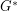by assigning each edge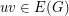for whicha sign ofand every other edge ofsign. It is straightforward to verify that the restriction of any new eigenvector oftowill then be an eigenvector of. Thus, the above conjecture is equivalent to the conjecture that every-regular graph has a-lift so that all new eigenvalues have magnitude at most. Furthermore, a positive solution to this conjecture for-regular Ramanujan graphs would yield families of-regular expanders.

## Bibliography

*[BL] Y. Bilu, N. Linial, Lifts, discrepancy and nearly optimal spectral gap, Combinatorica 26 (5) (2006) 495–519. MathSciNet

[F] J. Friedman, Relative expanders or weakly relatively Ramanujan graphs, Duke Math. J. 118 (1) (2003) 19–35. MathSciNet

[LP] N. Linial, D. Puder, Word maps and spectra of random graph lifts, Random Structures Algorithms 37 (1) (2010) 100–135. MathSciNet

[LSV] E. Lubetzky, B. Sudakov, V Vu, Spectra of lifted Ramanujan graphs. Adv. Math. 227 (2011), no. 4, 1612–1645. MathSciNet

* indicates original appearance(s) of problem.